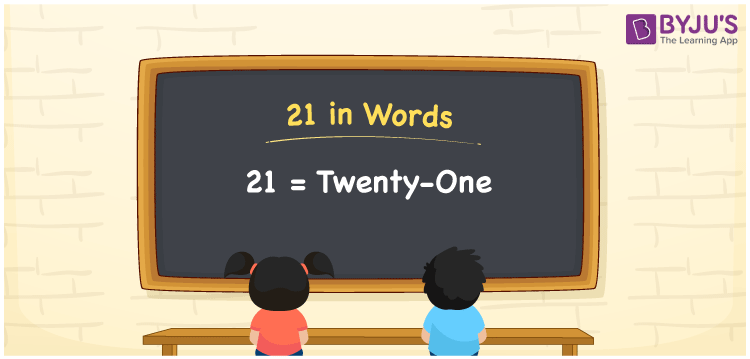# 21 in Words

21 in words can be written as Twenty-one. With the help of the article 21 in words, students will get a firm knowledge of the fundamentals of counting. For example, if you buy 1 kg tomato for Rs. 21, then you can say that “I bought 1 kg tomato for Twenty-one Rupees”. Using this article, you will get to know how to convert 21 into words. You can write numbers in words using the English alphabet. You can read 21 as “Twenty-one” in English.

 21 in words Twenty-one Twenty-one in Numbers 21

## 21 in English Words## How to Write 21 in Words?

The topic of writing 21 in words using the place value chart can be learnt in this section. The detailed explanation given here helps students get a good hold on the basic concepts of counting numbers. 21 is a two digit number and the place value chart for the number is provided in a table form here.

 Tens Ones 2 1

21 in expanded form is discussed here:

2 × Ten + 1 × One

= 2 × 10 + 1 × 1

= 20 + 1

= 21

= Twenty-one

Therefore, 21 in words is written as Twenty-one.

21 is a natural number that precedes 22 and succeeds 20.

21 in words – Twenty-one

Is 21 an odd number? – Yes

Is 21 an even number? – No

Is 21 a perfect square number? – No

Is 21 a perfect cube number? – No

Is 21 a prime number? – No

Is 21 a composite number? – Yes

## Frequently Asked Questions on 21 in Words

Q1

### How can the number 21 be written in words?

21 can be written in words as “Twenty-one”.
Q2

### How to write 21 on a cheque?

21 can be written on a cheque as Twenty-one rupees only.
Q3

### Is 21 an odd number?

21 is an odd number as it cannot be divided by 2.